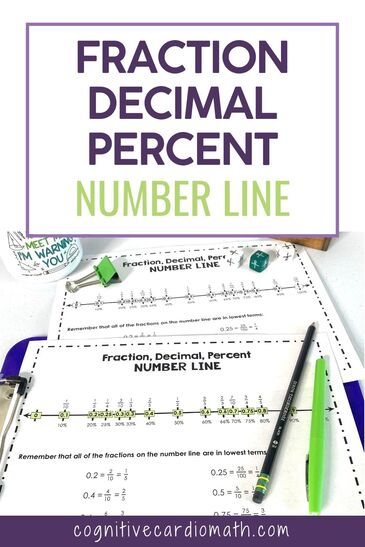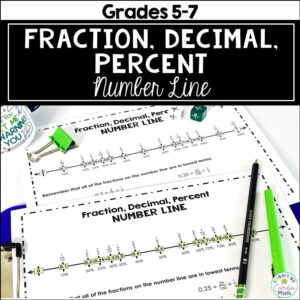# Fraction, Decimal, and Percent Conversions

## Classroom Fraction, Decimal Percent Number LineDo your middle school math students need reinforcement of fraction, decimal, percent conversions and equivalences? Every year, my math students needed extra reinforcement of the most common equivalences.

So, a few years ago, I made a number line with common decimals between 0 and 1 as the main number line. I added the equivalent fractions above the decimals and the equivalent percentages below them.

I keep this number line above the whiteboard all year long (except during state testing, of course!) in the hopes that by the time we get to this unit, some of these equivalences may have stuck in some students’ brains:-)

Does it work? I think it does…for some. However, for too many math students, I think it might be invisible until it can help them with their homework, lol.

The nice thing is that once students do realize the number line can help them, I notice them looking at it quite often. Even after the unit is “over,” they continue to use this number line as a resource.

## New Fraction, Decimal, Percent Number Lines

On my snow day the other day, I decided to make  a smaller version of the number line for the students to keep as a resource in their math binders (I added the fraction 2/3 to the student version…missed it on the larger one).

I’ve also created smaller number lines for the students to use as bookmarks – in the math books or in the books they read for pleasure! This gives them super easy access to these equivalences:-)

Need another resource to help teach fraction, decimal, conversions? Check out the Fraction, Decimal, Percent Math Wheel post!## EllieWelcome to Cognitive Cardio Math! I’m Ellie, a wife, mom, grandma, and dog ‘mom,’ and I’ve spent just about my whole life in school! With nearly 30 years in education, I’ve taught:

• All subject areas in 4th and 5th grades
• Math, ELA, and science in 6th grade (middle school)

I’ve been creating resources for teachers since 2012 and have worked in the elearning industry for about five years as well!